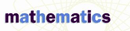# Angles of Elevation and Depression - Research Article from Macmillan Science Library: Mathematics

This encyclopedia article consists of approximately 4 pages of information about Angles of Elevation and Depression.
 This section contains 924 words (approx. 4 pages at 300 words per page) View a FREE sampleAngles of elevation and depression are angles that are formed with the horizontal. If the line of sight is upward from the horizontal, the angle is an angle of elevation; if the line of sight is downward from the horizontal, the angle is an angle of depression. These types of angles and some trigonometry can be used to indirectly calculate heights of objects or distances between points. Alternatively, if the heights or distances are known, the angles can be determined.

## Angles of Elevation

Finding a Flagpole's Height. Suppose that the height of a flagpole must be determined. The flagpole forms a right angle with level ground. Suppose the flagpole casts a shadow of 20 feet. See part (a) of the figure on the following page. The 20-foot shadow and the flagpole itself form two legs of a right triangle. The triangle's...

 This section contains 924 words (approx. 4 pages at 300 words per page) View a FREE sample